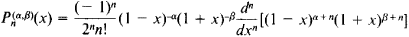# Rodrigues Formula

## Rodrigues formula

[rə′drē·gəs ‚fȯr·myə·lə]
(mathematics)
The equation giving the n th function in a class of special functions in terms of the n th derivatives of some polynomial.
The formula d n+ k d r= 0, expressing the difference d nin the unit normals to a surface at two neighboring points on a line of curvature, in terms of the difference d rin the position vectors of the two points and the principal curvature k.
The formula for a matrix that is used to transform the cartesian coordinates of a vector in three-space under a rotation through a specified angle about an axis with specified direction cosines.

## Rodrigues Formula

the expression for Legendre polynomialsThis formula was derived by the French mathematician B. O. Rodrigues in 1814. In 1859 the German mathematician K. Jacobi extended the formula to the case of Jacobi polynomials. In this case, it has the formThe Rodrigues formula can be incorporated into the theory of Legendre and Jacobi polynomials; in particular, by using the formula it is a simple matter to derive the basic properties of these polynomials. It also follows from the formula that these polynomials are special cases of the hypergeometric function.

Mentioned in ?
References in periodicals archive ?
Basic properties, such as a Rodrigues formula, a differential equation, recurrence relations and an orthogonality relation are derived.
Taking into account (5), it is easily seen that there also exists a Rodrigues formula.
For these Gegenbauer polynomials associated with Hk we can also derive a Rodrigues formula, a differential equation, an annihilation equation and a recurrence relation.

Site: Follow: Share:
Open / Close